# 数据结构--最小生成树详解

A wise man changes his mind,a fool never.
Name:Willam
Time:2017/3/1

## 2、普里姆算法—Prim算法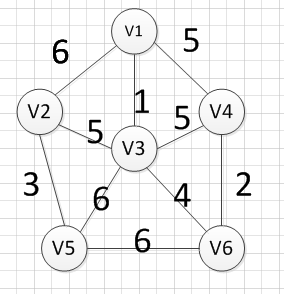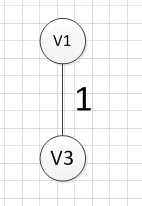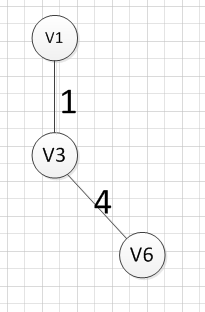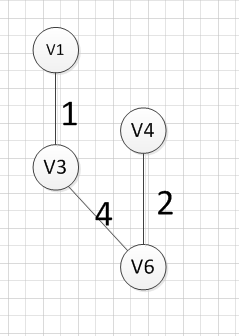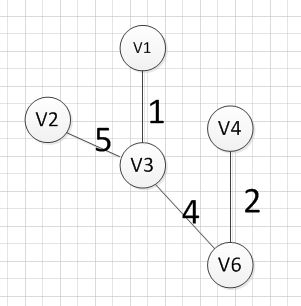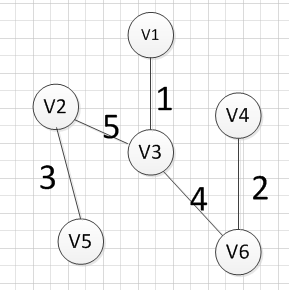## 3、普里姆算法—代码实现

（1）采用的是邻接矩阵的方式存储图，代码如下

#include<iostream>
#include<string>
#include<vector>
using  namespace std;

//首先是使用邻接矩阵完成Prim算法
struct Graph {
int vexnum;  //顶点个数
int edge;   //边的条数
int ** arc; //邻接矩阵
string *information; //记录每个顶点名称
};

//创建图
void createGraph(Graph & g) {
cout << "请输入顶点数：输入边的条数" << endl;
cin >> g.vexnum;
cin >> g.edge;  //输入边的条数

g.information = new string[g.vexnum];
g.arc = new int*[g.vexnum];
int i = 0;

//开辟空间的同时，进行名称的初始化
for (i = 0; i < g.vexnum; i++) {
g.arc[i] = new int[g.vexnum];
g.information[i]="v"+ std::to_string(i+1);//对每个顶点进行命名
for (int k = 0; k < g.vexnum; k++) {
g.arc[i][k] = INT_MAX;          //初始化我们的邻接矩阵
}
}

cout << "请输入每条边之间的顶点编号(顶点编号从1开始),以及该边的权重：" << endl;
for (i = 0; i < g.edge; i++) {
int start;
int end;
cin >> start;   //输入每条边的起点
cin >> end;     //输入每条边的终点
int weight;
cin >> weight;
g.arc[start-1][end-1]=weight;//无向图的边是相反的
g.arc[end-1][start-1] = weight;
}
}

//打印图
void print(Graph g) {
int i;
for (i = 0; i < g.vexnum; i++) {
//cout << g.information[i] << " ";
for (int j = 0; j < g.vexnum; j++) {
if (g.arc[i][j] == INT_MAX)
cout << "∞" << " ";
else
cout << g.arc[i][j] << " ";
}
cout << endl;
}
}

//作为记录边的信息，这些边都是达到end的所有边中，权重最小的那个
struct Assis_array {
int start; //边的终点
int end;  //边的起点
int weight;  //边的权重
};
//进行prim算法实现，使用的邻接矩阵的方法实现。
void Prim(Graph g,int begin) {

//close_edge这个数组记录到达某个顶点的各个边中的权重最大的那个边
Assis_array *close_edge=new Assis_array[g.vexnum];

int j;

//进行close_edge的初始化，更加开始起点进行初始化
for (j = 0; j < g.vexnum; j++) {
if (j != begin - 1) {
close_edge[j].start = begin-1;
close_edge[j].end = j;
close_edge[j].weight = g.arc[begin - 1][j];
}
}
//把起点的close_edge中的值设置为-1，代表已经加入到集合U了
close_edge[begin - 1].weight = -1;
//访问剩下的顶点，并加入依次加入到集合U
for (j = 1; j < g.vexnum; j++) {

int min = INT_MAX;
int k;
int index;
//寻找数组close_edge中权重最小的那个边
for (k = 0; k < g.vexnum; k++) {
if (close_edge[k].weight != -1) {
if (close_edge[k].weight < min) {
min = close_edge[k].weight;
index = k;
}
}
}
//将权重最小的那条边的终点也加入到集合U
close_edge[index].weight = -1;
//输出对应的边的信息
cout << g.information[close_edge[index].start]
<< "-----"
<< g.information[close_edge[index].end]
<< "="
<<g.arc[close_edge[index].start][close_edge[index].end]
<<endl;

//更新我们的close_edge数组。
for (k = 0; k < g.vexnum; k++) {
if (g.arc[close_edge[index].end][k] <close_edge[k].weight) {
close_edge[k].weight = g.arc[close_edge[index].end][k];
close_edge[k].start = close_edge[index].end;
close_edge[k].end = k;
}
}
}
}

int main()
{
Graph g;
createGraph(g);//基本都是无向网图，所以我们只实现了无向网图
print(g);
Prim(g, 1);
system("pause");
return 0;
}

6 10
1 2 6
1 3 1
1 4 5
2 3 5
2 5 3
3 5 6
3 6 4
4 3 5
4 6 2
5 6 6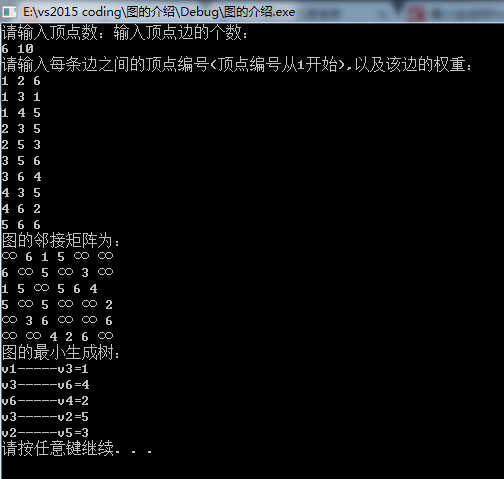（2）采用的是邻接表的方式存储图，代码如下

#include<iostream>
#include<string>
using  namespace std;
//表结点
struct ArcNode {
ArcNode * next;  //指向下一个表结点
int weight;      //边的权重
};
//头结点
struct Vnode {
ArcNode * firstarc;  //第一个和该顶点依附的边 的信息
string data;       //记录该顶点的信息。
};

struct Graph_List {
int vexnum;     //顶点个数
int edge;       //边的条数
Vnode * node;  //顶点表
};

//创建图，是一个重载函数
void createGraph(Graph_List &g) {
cout << "请输入顶点数：输入顶点边的个数：" << endl;
cin >> g.vexnum;
cin >> g.edge;
g.node = new Vnode[g.vexnum];
int i;
for (i = 0; i < g.vexnum; i++) {
g.node[i].data = "v" + std::to_string(i + 1);  //对每个顶点进行命名
g.node[i].firstarc = NULL;//初始化每个顶点的依附表结点
}

cout << "请输入每条边之间的顶点编号(顶点编号从1开始),以及该边的权重：" << endl;
for (i = 0; i < g.edge; i++) {
int start;
int end;
cin >> start;   //输入每条边的起点
cin >> end;     //输入每条边的终点
int weight;
cin >> weight;

ArcNode * next = new ArcNode;
next->next = NULL;
next->weight = weight;
//如果第一个依附的边为空
if (g.node[start - 1].firstarc == NULL) {
g.node[start - 1].firstarc = next;
}
else {
ArcNode * temp; //临时表结点
temp = g.node[start - 1].firstarc;
while (temp->next) {//找到表结点中start-1这个结点的链表的最后一个顶点
temp = temp->next;
}
temp->next = next;  //在该链表的尾部插入一个结点

}
//因为无向图边是双向的
ArcNode * next_2 = new ArcNode;
next_2->weight = weight;
next_2->next = NULL;

//如果第一个依附的边为空
if (g.node[end - 1].firstarc == NULL) {
g.node[end - 1].firstarc = next_2;
}
else {
ArcNode * temp; //临时表结点
temp = g.node[end - 1].firstarc;
while (temp->next) {//找到表结点中start-1这个结点的链表的最后一个顶点
temp = temp->next;
}
temp->next = next_2;  //在该链表的尾部插入一个结点

}

}
}

void print(Graph_List g) {
cout<<"图的邻接表："<<endl;
for (int i = 0; i < g.vexnum; i++) {
cout << g.node[i].data << " ";
ArcNode * next;
next = g.node[i].firstarc;
while (next) {
cout << "("<< g.node[i].data <<","<<g.node[next->adjvex].data<<")="<<next->weight << " ";
next = next->next;
}
cout << "^" << endl;
}
}

////作为记录边的信息，这些边都是达到end的所有边中，权重最小的那个
struct Assis_array {
int start; //边的终点
int end;  //边的起点
int weight;  //边的权重
};

void Prim(Graph_List g, int begin) {
cout << "图的最小生成树：" << endl;
//close_edge这个数组记录到达某个顶点的各个边中的权重最大的那个边
Assis_array *close_edge=new Assis_array[g.vexnum];
int j;
for (j = 0; j < g.vexnum; j++) {
close_edge[j].weight = INT_MAX;
}
ArcNode * arc = g.node[begin - 1].firstarc;

while (arc) {
arc = arc->next;
}
//把起点的close_edge中的值设置为-1，代表已经加入到集合U了
close_edge[begin - 1].weight = -1;
//访问剩下的顶点，并加入依次加入到集合U
for (j = 1; j < g.vexnum; j++) {
int min = INT_MAX;
int k;
int index;
//寻找数组close_edge中权重最小的那个边
for (k = 0; k < g.vexnum; k++) {
if (close_edge[k].weight != -1) {
if (close_edge[k].weight < min) {
min = close_edge[k].weight;
index = k;
}
}
}

//输出对应的边的信息
cout << g.node[close_edge[index].start].data
<< "-----"
<< g.node[close_edge[index].end].data
<< "="
<< close_edge[index].weight
<<endl;
//将权重最小的那条边的终点也加入到集合U
close_edge[index].weight = -1;
//更新我们的close_edge数组。
ArcNode * temp = g.node[close_edge[index].end].firstarc;
while (temp) {
}
temp = temp->next;
}
}

}
int main()
{
Graph_List g;
createGraph(g);
print(g);
Prim(g, 1);
system("pause");
return 0;


6 10
1 2 6
1 3 1
1 4 5
2 3 5
2 5 3
3 5 6
3 6 4
4 3 5
4 6 2
5 6 6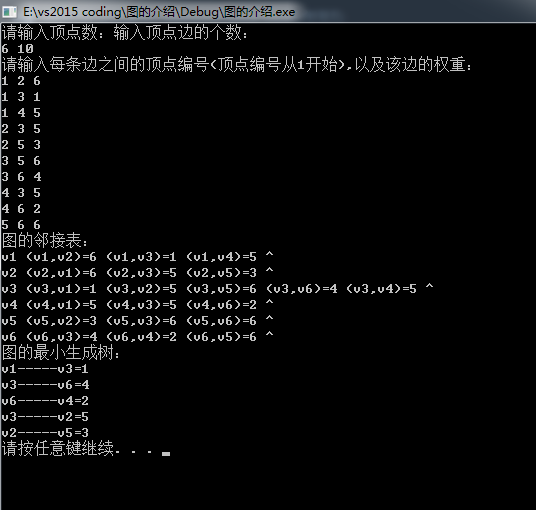## 4、克鲁斯卡算法

（1）将图中的所有边都去掉。
（2）将边按权值从小到大的顺序添加到图中，保证添加的过程中不会形成环
（3）重复上一步直到连接所有顶点，此时就生成了最小生成树。这是一种贪心策略。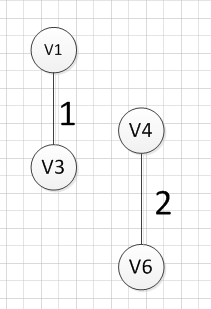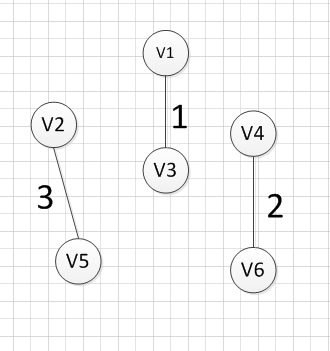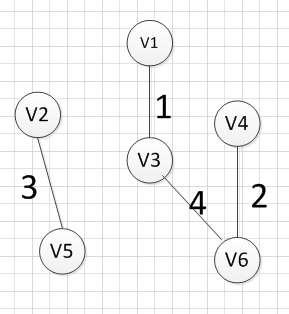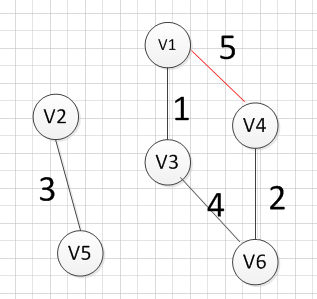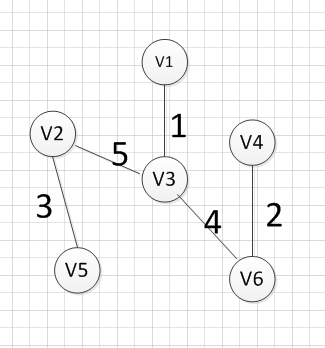OK，到这里，我们已经把克鲁斯卡算法过了一遍，下面我们就用具体的代码实现它：

## 5、克鲁斯卡算法的代码实现

/************************************************************/
/*                程序作者：Willam                          */
/*                程序完成时间：2017/3/3                    */
/*                有任何问题请联系：2930526477@qq.com       */
/************************************************************/
//@尽量写出完美的程序

#include<iostream>
#include<algorithm>
#include<string>
using namespace std;

//检验输入边数和顶点数的值是否有效，可以自己推算为啥：
//顶点数和边数的关系是：((Vexnum*(Vexnum - 1)) / 2) < edge
bool check(int Vexnum,int edge) {
if (Vexnum <= 0 || edge <= 0 || ((Vexnum*(Vexnum - 1)) / 2) < edge)
return false;
return true;
}

//判断我们每次输入的的边的信息是否合法
//顶点从1开始编号
bool check_edge(int Vexnum, int start ,int end, int weight) {
if (start<1 || end<1 || start>Vexnum || end>Vexnum || weight < 0) {
return false;
}
return true;
}

//边集结构，用于保存每条边的信息
typedef struct edge_tag {
bool visit; //判断这条边是否加入到了最小生成树中
int start;   //该边的起点
int end;   //该边的终点
int weight; //该边的权重
}Edge;

//创建一个图，但是图是使用边集结构来保存
void createGraph(Edge * &e,int Vexnum, int edge) {
e = new Edge[edge];//为每条边集开辟空间
int start = 0;
int end = 0;
int weight = 0;

int i = 0;
cout << "输入每条边的起点、终点和权重：" << endl;
while (i != edge)
{
cin >> start >> end >> weight;
while (!check_edge(Vexnum, start, end, weight)) {
cout << "输入的值不合法，请重新输入每条边的起点、终点和权重：" << endl;
cin >> start >> end >> weight;
}
e[i].start = start;
e[i].end = end;
e[i].weight = weight;
e[i].visit = false; //每条边都还没被初始化
++i;

}
}

//我们需要对边集进行排序，排序是按照每条边的权重，从小到大排序。
int cmp(const void*  first, const void * second) {
return ((Edge *)first)->weight - ((Edge *)second)->weight;
}

//好了，我们现在需要做的是通过一定的方式来判断
//如果我们把当前的边加入到生成树中是否会有环出现。
//通过我们之前学习树的知识，我们可以知道如果很多棵树就组成一个森林，而且
//如果同一颗树的两个结点在连上一条边，那么就会出现环，
//所以我们就通过这个方式来判断加入了一个新的边后，是否会产生环，
//开始我们让我们的图的每个顶点都是一颗独立的树，通过不断的组合，把这个森林变
//成来源于同一颗顶点的树
//如果不理解，画个图就明白了，

//首先是找根节点的函数,
//其中parent代表顶点所在子树的根结点
//child代表每个顶点孩子结点的个数
int find_root(int child, int * parent) {

//此时已经找到了该顶点所在树的根节点了
if (parent[child] == child) {
return child;
}
//往前递归，寻找它父亲的所在子树的根结点
parent[child] = find_root(parent[child], parent);
return parent[child];
}

//合并两个子树
bool union_tree(Edge  e, int * parent, int * child) {
//先找出改边所在子树的根节点
int root1;
int root2;
//记住我们顶点从1开始的，所以要减1
root1 = find_root(e.start-1, parent);
root2 = find_root(e.end-1, parent);
//只有两个顶点不在同一颗子树上，才可以把两棵树并未一颗树
if (root1 != root2) {
//小树合并到大树中，看他们的孩子个数
if (child[root1] > child[root2]) {
parent[root2] = root1;
//大树的孩子数量是小树的孩子数量加上
//大树的孩子数量在加上小树根节点自己
child[root1] += child[root2] + 1;
}
else {
parent[root1] = root2;
child[root2] += child[root1] + 1;
}
return true;
}
return false;
}

//克鲁斯卡算法的实现
void Kruskal() {
int Vexnum = 0;
int edge = 0;
cout << "请输入图的顶点数和边数：" << endl;
cin >> Vexnum >> edge;
while (!check(Vexnum, edge)) {
cout << "你输入的图的顶点数和边数不合法，请重新输入：" << endl;
cin >> Vexnum >> edge;
}

//声明一个边集数组
Edge * edge_tag;
//输入每条边的信息
createGraph(edge_tag, Vexnum, edge);

int * parent = new int[Vexnum]; //记录每个顶点所在子树的根节点下标
int * child = new int[Vexnum]; //记录每个顶点为根节点时，其有的孩子节点的个数
int i;
for (i = 0; i < Vexnum; i++) {
parent[i] = i;
child[i] = 0;
}
//对边集数组进行排序，按照权重从小到达排序
qsort(edge_tag, edge, sizeof(Edge), cmp);
int count_vex; //记录输出的边的条数

count_vex = i = 0;
while (i != edge) {
//如果两颗树可以组合在一起，说明该边是生成树的一条边
if (union_tree(edge_tag[i], parent, child)) {
cout << ("v" + std::to_string(edge_tag[i].start))
<< "-----"
<< ("v" + std::to_string(edge_tag[i].end))
<<"="
<< edge_tag[i].weight
<< endl;
edge_tag[i].visit = true;
++count_vex; //生成树的边加1
}
//这里表示所有的边都已经加入成功
if (count_vex == Vexnum - 1) {
break;
}
++i;
}

if (count_vex != Vexnum - 1) {
cout << "此图为非连通图！无法构成最小生成树。" << endl;
}
delete [] edge_tag;
delete [] parent;
delete [] child;
}

int main() {
Kruskal();
system("pause");
return 0;
}

6 10
1 2 6
1 3 1
1 4 5
2 3 5
2 5 3
3 5 6
3 6 4
4 3 5
4 6 2
5 6 6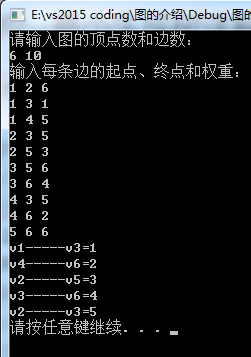7 9
1 2 20
1 5 1
2 3 6
2 4 4
3 7 2
4 6 12
4 7 8
5 6 15
6 7 10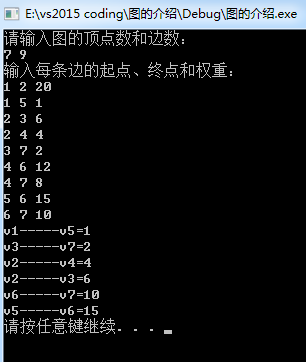04-22320

#### 【图论】最小生成树（Prim 和 Kruskal算法）

08-073万+

#### 最小生成树（kruskal算法）

11-011万+

#### 图——最小生成树

05-11979

#### 最小生成树

11-121872

#### 【图(下)】最小生成树问题

07-149万+

#### 算法导论--最小生成树（Kruskal和Prim算法）

10-302万+

#### 最小生成树（普里姆算法）

12-239264

#### 最小生成树的两种方法

11-041682

#### 最小生成树-Kruscal算法

04-06456

#### 数据结构--最小生成树详解与实现

09-171万+

#### 最小生成树：Prim算法

09-2747万+

#### 最新微信小程序源码

05-237997

#### 微信小程序源码-合集1

03-25694

#### X-CUBE-MCSDK-FUL_5.4.3.rar

01-07

02-245971

#### 数据结构---二叉树的详解

08-1612万+

#### 最小生成树Prim算法理解

11-152万+

#### 数据结构--线性表详解（一）

03-267231

#### 二叉排序树详解

11-12862

#### 【学习笔记----数据结构19-图的最小生成树】

03-263万+

#### “程序员数学不行，干啥都不行！”高级开发：90%都是瞎努力！

08-2727

#### 浅谈数据结构-最小生成树

02-213万+

#### 最小生成树最小生成树最小生成树最小生成树

12-06

03-074万+

#### 数据结构----关键路径详解

11-19277

#### 数据结构与算法之------最小生成树

04-03549

#### 最小生成树-贪心算法

04-10399

#### 数据结构 最小生成树（prime算法）

10-051025

#### 数据结构——图（8）——最小生成树（MST）

02-272440

#### 数据结构(十七)最小生成树

02-281万+

#### 数据结构----BFS和DFS详解

03-282万+

#### B-树的详解

07-16269

#### 【数据结构】最小生成树问题

11-152万+

#### 几分钟搞明白生成树和最小生成树的定义

03-07162

#### 最小生成树详解

04-284224

#### 6 步快速构建 Kubernetes 平台

03-252万+

#### 当HR压你价，说你只值7K，你该怎么回答？

04-092万+

#### 代码注释如此沙雕，会玩还是你们程序员！

03-066万+

#### 数据结构---拓扑排序详解

03-18640

#### (三)什么是生成树，生成树（生成森林）详解

08-101233

#### 数据结构--图--最小生成树

02-212万+

#### 数据结构---串的模式匹配算法介绍

04-066万+

#### 终于，月薪过5万了！

03-243万+

#### 为什么程序员做外包会被瞧不起？

03-224万+

#### 男生更看重女生的身材脸蛋，还是思想？

03-263万+

#### 终于懂了TCP和UDP协议区别

02-1518万+

#### 在三线城市工作爽吗？

10-24617

#### 51nod 1212 无向图最小生成树 （Kruskal）

07-152万+

#### 【算法】图的最小生成树(Kruskal算法)

12-309523

#### 最小生成树算法总结

03-233万+

#### 程序员毕业去大公司好还是小公司好？

04-302万+

#### 如何优雅的替换掉代码中的ifelse

03-274万+

#### 无代码时代来临，程序员如何保住饭碗？

06-261万+

#### 最小生成树怎么画？

03-231万+

#### 曾经废寝忘食学到的技术，现在都没用了......

03-193万+

#### 2020阿里全球数学大赛：3万名高手、4道题、2天2夜未交卷

05-084万+

#### 面试：第十六章：Java中级开发（16k）

08-07124

#### 数据结构之图(七)——最小生成树

10-2911万+

#### 中国麻将：世界上最早的区块链项目

03-055万+

#### 讲一个程序员如何副业月赚三万的真实故事

05-164788

#### 什么是单点登录(SSO)

02-02227

#### 【数据结构】 最小生成树（一）——什么是最小生成树？

03-203万+

#### 【Java面试题】List如何一边遍历，一边删除？

10-2818万+

#### 有哪些让程序员受益终生的建议

03-291万+

#### 0025算法笔记——【贪心算法】最小生成树问题

11-044148

#### 最小生成树的简单例题

03-0837万+

#### 最短路径问题---Dijkstra算法详解

11-07557

#### 数据结构——最小生成树（C语言）

02-121456

#### 图的最小生成树——例题

10-31163

#### 最小生成树&次小生成树算法精讲

05-141万+

#### 用python打开电脑摄像头，并把图像传回qq邮箱【Pyinstaller打包】

04-075万+

#### 我说我懂多线程，面试官立马给我发了offer

12-291万+

#### 所有边权均不相同的无向图最小生成树是唯一的证明

09-132064

#### 最小生成树问题

08-09105

#### 关于最小生成树的一些理解©️2019 CSDN 皮肤主题: 大白 设计师: CSDN官方博客点击重新获取扫码支付